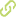# Vector addition: Effect of the context and position of the vectors•
• Overview
•
• Identity
•
•
• View All
•

### Abstract

• In this article we investigate the effect of: 1) the context, and 2) the position of the vectors, on 2D vector addition tasks. We administered a test to 512 students completing introductory physics courses at a private Mexican university. In the first part, we analyze students' responses in three isomorphic problems: displacements, forces, and no physical context. Students were asked to draw two vectors and the vector sum. We analyzed students' procedures detecting the difficulties when drawing the vector addition and proved that the context matters, not only compared to the context-free case but also between the contexts. In the second part, we analyze students' responses with three different arrangements of the sum of two vectors: tail-to-tail, head-to-tail and separated vectors. We compared the frequencies of the errors in the three different positions to deduce students' conceptions in the addition of vectors. © 2010 American Institute of Physics.

### Publication date

• December 1, 2010